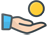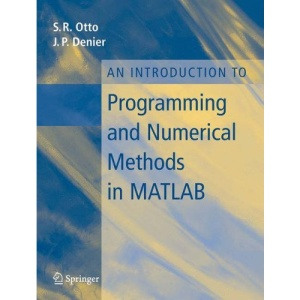30 Day Refund Guarantee# An Introduction to Programming and Numerical Methods in MATLAB

by StephenR.Otto (Author), JamesP.Denier (Author)

##### Synopsis

MATLAB is a programme which lends itself to the implementation of most numerical algorithms. This text, which uses MATLAB, gives an overview of structured programming and numerical methods and covers numerical methods for solving a range of problems, from integration to the numerical solution of differential equations.

Quantity

10 in stock